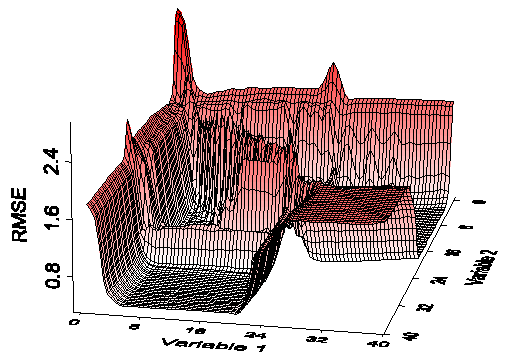Home News About Me Ph. D. Thesis Abstract Table of Contents 1. Introduction 2. Theory  Fundamentals of the Multivariate Data Analysis 2.1. Overview of the Multivariate Quantitative Data Analysis 2.2. Experimental Design 2.3. Data Preprocessing 2.4. Data Splitting and Validation 2.5. Calibration of Linear Relationships 2.6. Calibration of Nonlinear Relationships 2.7. Neural Networks  Universal Calibration Tools 2.8. Too Much Information Deteriorates Calibration 2.8.1. Overfitting, Underfitting and Model Complexity 2.8.2. Neural Networks and the Complexity Problem 2.8.3. Brute Force Variable Selection 2.8.4. Variable Selection by Stepwise Algorithms 2.8.5. Variable Selection by Genetic Algorithms 2.8.6. Variable Selection by Simulated Annealing 2.8.7. Variable Compression by Principal Component Analysis 2.8.8. Topology Optimization by Pruning Algorithms 2.8.9. Topology Optimization by Genetic Algorithms 2.8.10. Topology Optimization by Growing Neural Network Algorithms 2.9. Measures of Error and Validation 3. Theory  Quantification of the Refrigerants R22 and R134a: Part I 4. Experiments, Setups and Data Sets 5. Results  Kinetic Measurements 6. Results  Multivariate Calibrations 7. Results  Genetic Algorithm Framework 8. Results  Growing Neural Network Framework 9. Results  All Data Sets 10. Results  Various Aspects of the Frameworks and Measurements 11. Summary and Outlook 12. References 13. Acknowledgements Publications Research Tutorials Downloads and Links Contact Search Site Map Print this Page### 2.8.4.   Variable Selection by Stepwise Algorithms

The two stepwise algorithms forward addition (forward selection) and backward elimination (backward selection) are also sometimes called gradient methods as the next addition or elimination step is performed on the basis of the steepest gradient of the error surface. The forward selection begins by selecting the variable, which results in the lowest error of prediction. In the next step, the variable out of the remaining variables is added, which minimizes the error in combination with the first variable. The stepwise addition of further variables is repeated until an optimal subset is found with a maximum of ntot steps. The backward elimination works in the opposite direction by starting with all variables and eliminating single variables. In addition, combinations of both methods are known as stepwise multiple regressions . Yet, the stepwise algorithms fail to take the information into account that involves the combined effect of several variables. Thus, these algorithms hardly find an optimal solution, which requires several independent variables to be selected ,. The stepwise algorithms walk during the minimum search in the valleys of the error surface and cannot find minima surrounded by high mountains. In figure 4, the error surface of the selection of 2 variables out of 40 is shown for the refrigerant data introduced in section 4.5.1.1. Even in this figure, which represents only a highly constrained 2-dimensional lateral surface of the 40-dimensional error surface, it is visible that the error surface is too rough for the stepwise algorithms finding an optimal solution and not usable for high dimensional data sets with many correlated variables.figure 4:    Root mean square of prediction versus the index of the 2 variables selected.

 Page 51 © Frank Dieterle, 03.03.2019